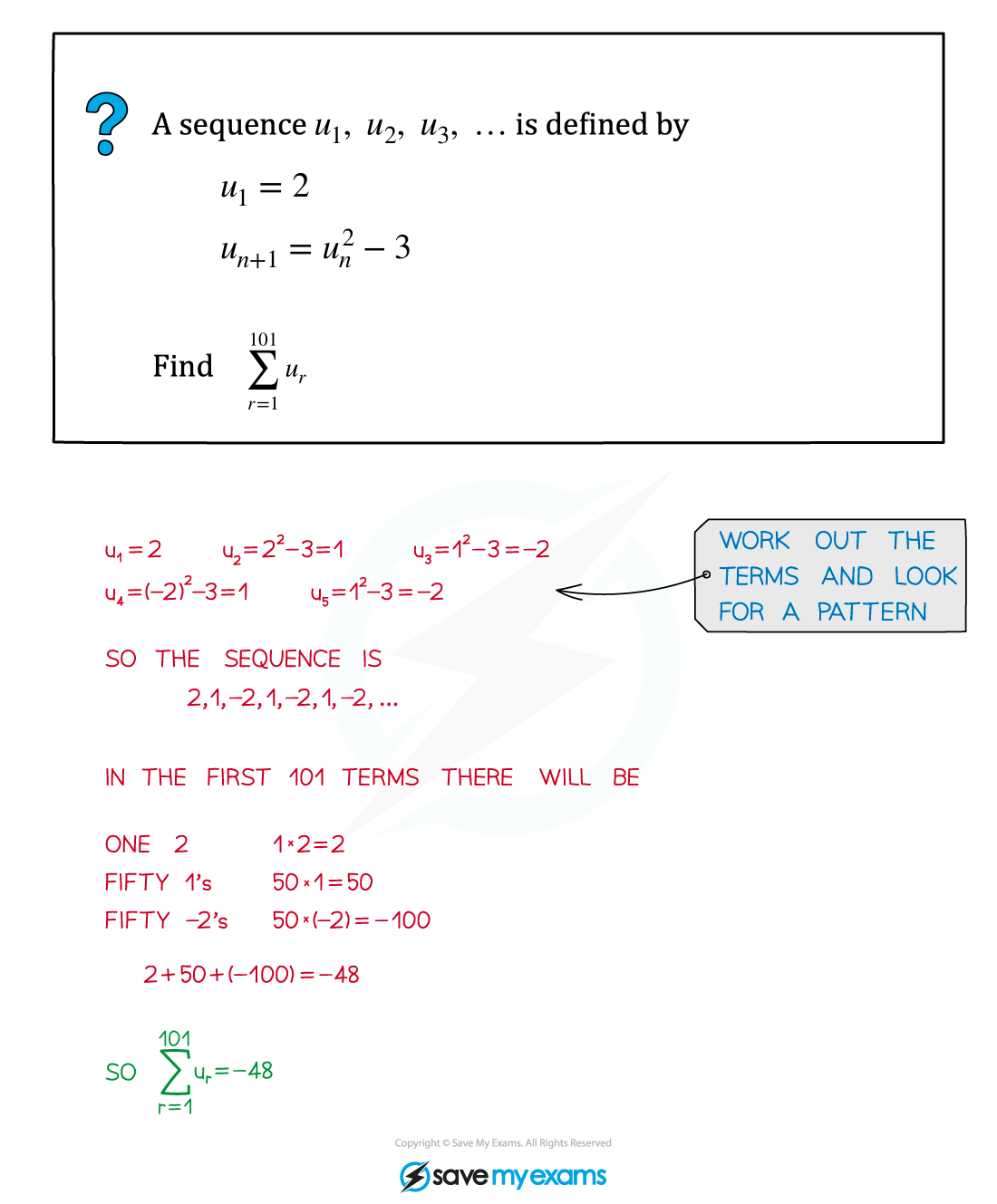# AQA A Level Maths: Pure复习笔记4.5.3 Recurrence Relations

### Recurrence Relations

#### What do I need to know about recurrence relations?

• A recurrence relation describes each term in a sequence as a function of the previous term – ie un+1 = f(un)
• Along with the first term of the sequence, this allows you to generate the sequence term by term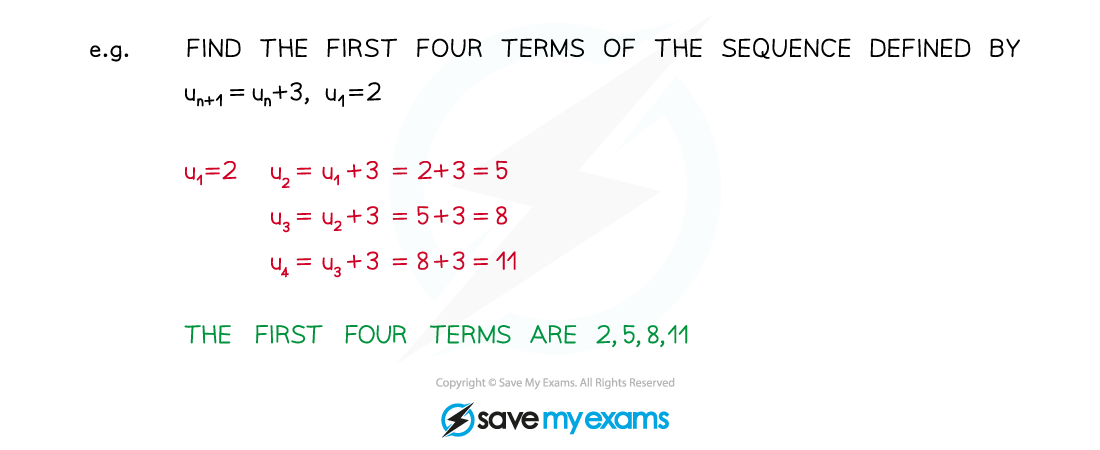• Both arithmetic sequences and geometric sequences can be defined using recurrence relations
•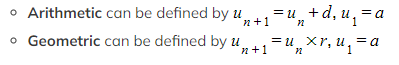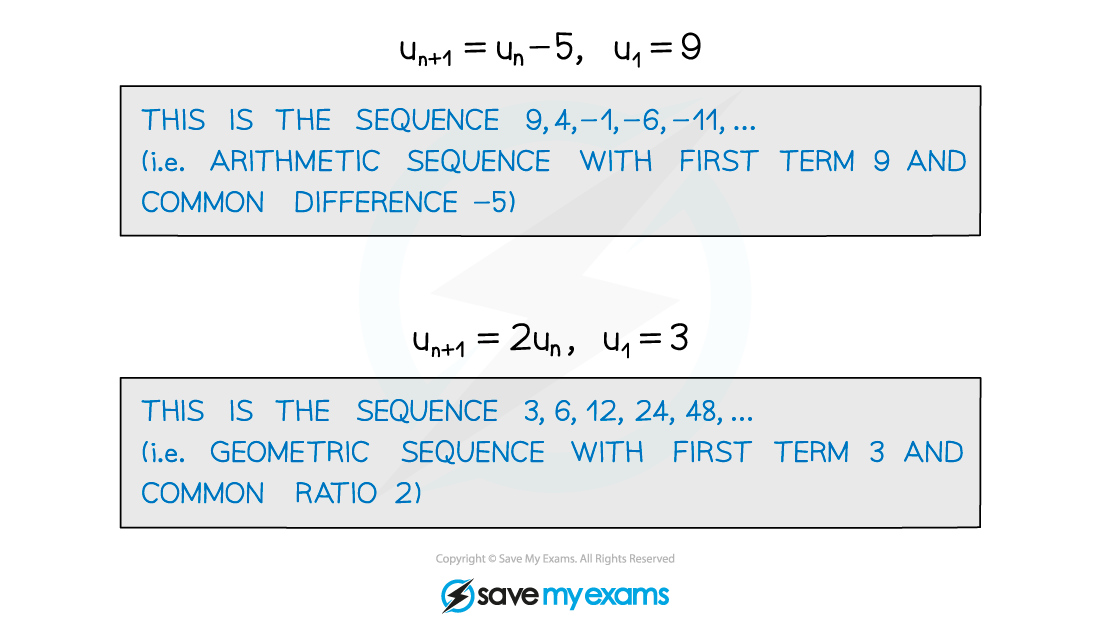• However, you can also define sequences that are neither arithmetic nor geometric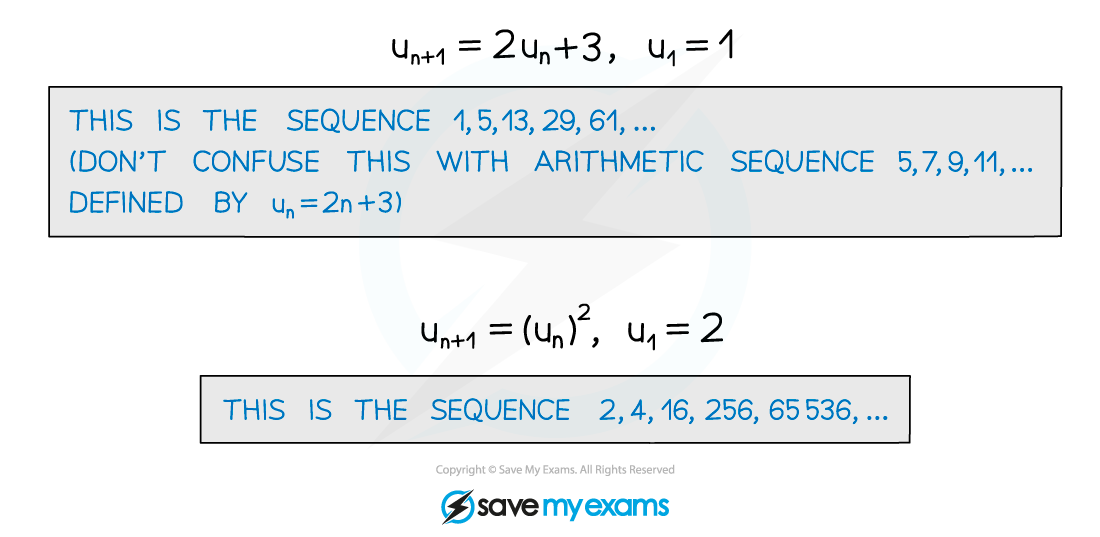#### Exam Tip

• For arithmetic or geometric sequences defined by recurrence relations, you can sum the terms using the arithmetic series and geometric series formulae.
• To sum up the terms of other sequences, you may have to think about the series and find a clever trick.

#### Worked Example